# C++ Program to Find the Area and Perimeter of a Rectangle

In this article, you will learn and get code for printing the area and perimeter of a rectangle based on length and breadth entered by the user at run-time in C++ programming. Here is the list of approaches used to find the area and perimeter of a rectangle:

### Area of a Rectangle Formula

The formula to calculate the area of a rectangle is given below::

`area = len*bre`

Here, len indicates length and bre indicates breadth of the rectangle.

### Perimeter of the Rectangle Formula

To calculate the perimeter of a rectangle, use the following formula:

`perimeter = 2*(len+bre)`

Here also, len and bre indicate the length and breadth of the rectangle, respectively. Now let's move on to the program.

## In C++, find the area of a rectangle

The question is, "Write a program in C++ to find and print the area of a rectangle." The program given below is the answer to this question:

```#include<iostream>
using namespace std;
int main()
{
float len, bre, area;
cout<<"Enter Length of Rectangle: ";
cin>>len;
cin>>bre;
area = len*bre;
cout<<"\nArea = "<<area;
cout<<endl;
return 0;
}```

This program was built and runs under the Code::Blocks IDE. Here is its sample run: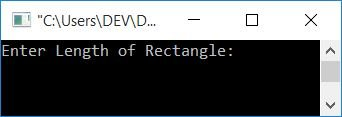Now enter the length and breadth of the rectangle and press the ENTER key to see the area based on the given length and breadth of the rectangle, as shown in the output given below: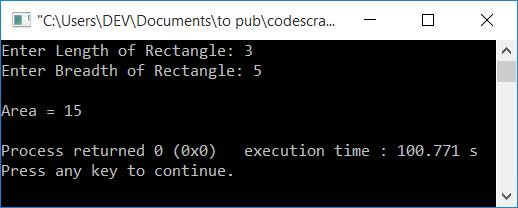When the user enters length, it gets initialized to len, and when the user enters breadth, it gets initialized to bre. Using the formula, len*bre gets initialized to the area variable, which holds the area of the rectangle. Therefore, just print its value as output.

### Using the function to calculate the area of a rectangle

Let's create the same-purpose program using a user-defined function, areaOfRectangle(). This function takes two arguments and returns a single value. The two arguments are length and breadth, whereas its return value will be the area of the rectangle.

```#include<iostream>
using namespace std;
float areaOfRectangle(float, float);
int main()
{
float len, bre, area;
cout<<"Enter Length of Rectangle: ";
cin>>len;
cin>>bre;
area = areaOfRectangle(len, bre);
cout<<"\nArea = "<<area;
cout<<endl;
return 0;
}
float areaOfRectangle(float len, float bre)
{
return (len*bre);
}```

## In C++, determine the perimeter of a rectangle

Now let's find and print the perimeter of a rectangle without using a function.

```#include<iostream>
using namespace std;
int main()
{
float len, bre, per;
cout<<"Enter Length and Breadth of Rectangle: ";
cin>>len>>bre;
per = 2*(len+bre);
cout<<"\nPerimeter = "<<per;
cout<<endl;
return 0;
}```

Here is a sample run of the above program: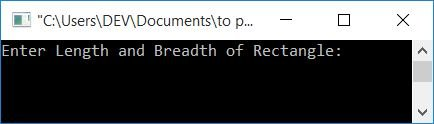Now supply the values of length and breadth of a rectangle to find its perimeter and print it as shown in the output given below.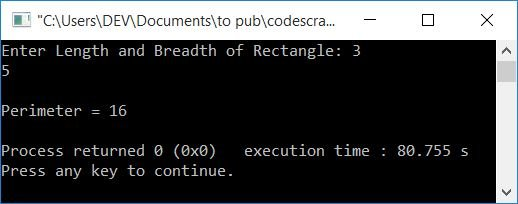### Perimeter of a rectangle using function

Here is another program that also finds and prints the perimeter of a rectangle using a user-defined function.

```#include<iostream>
using namespace std;
float perOfRectangle(float, float);
int main()
{
float len, bre;
cout<<"Enter Length and Breadth of Rectangle: ";
cin>>len>>bre;
cout<<"\nPerimeter = "<<perOfRectangle(len, bre);
cout<<endl;
return 0;
}
float perOfRectangle(float len, float bre)
{
return (2*(len+bre));
}```

## Using Class, calculate the area and perimeter of a rectangle

Now let's combine both and create a single program using classes to find the area and perimeter of a rectangle.

```#include<iostream>
using namespace std;
class CodesCracker
{
private:
float len, bre;
public:
void getData();
float areaOfRect();
float periOfRect();
};
void CodesCracker::getData()
{
cout<<"Enter Length and Breadth of Rectangle: ";
cin>>len>>bre;
}
float CodesCracker::areaOfRect()
{
return (len*bre);
}
float CodesCracker::periOfRect()
{
return (2*(len+bre));
}
int main()
{
CodesCracker c;
float area, per;
c.getData();
area = c.areaOfRect();
per = c.periOfRect();
cout<<"\nArea = "<<area;
cout<<"\nPerimeter = "<<per;
cout<<endl;
return 0;
}```

Here is its sample run with user input of 5 as length and 3 as breadth: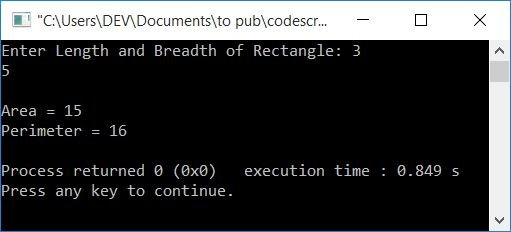C++ Quiz

« Previous Program Next Program »